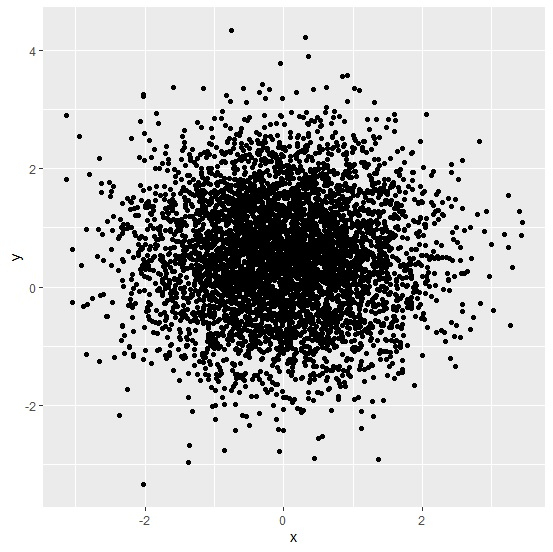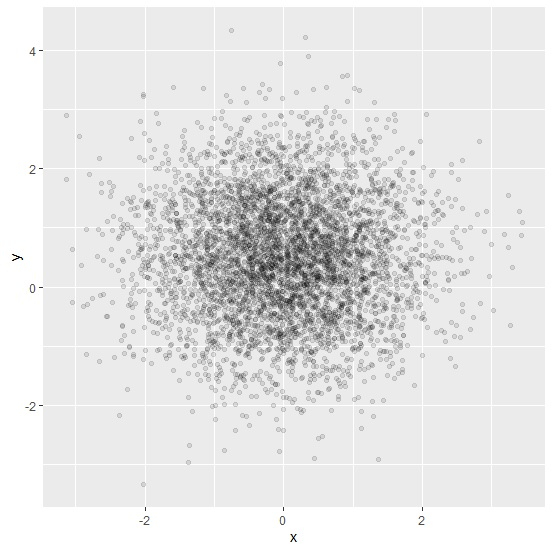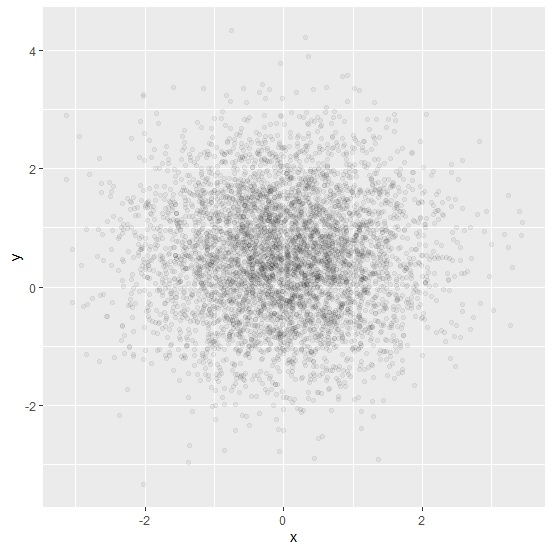# How to create a scatterplot in R using ggplot2 with transparency of points?

A scatterplot is used to observe the relationship between two continuous variables. If the sample size is large then the points on the plot lie on each other and does not look appealing. Also, the interpretation of such type of scatterplots is not an easy task, therefore, we can increase the transparency of points on the plot to make it more appealing. We can do this by using alpha argument in geom_point of ggplot2.

## Example

Consider the below data frame −

> set.seed(123)
> x<-rnorm(5000)
> y<-rnorm(5000,0.5)
> df<-data.frame(x,y)
> library(ggplot2)
> ggplot(df,aes(x,y))+geom_point()

## Output> ggplot(df,aes(x,y))+geom_point(alpha=0.10)

## Output> ggplot(df,aes(x,y))+geom_point(alpha=0.05)

## Output